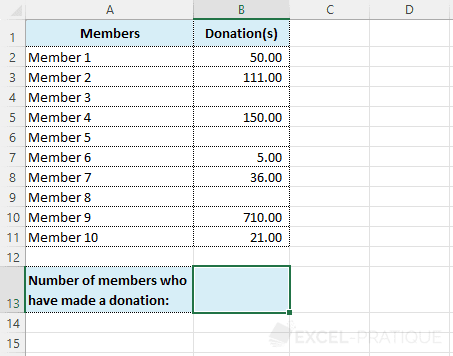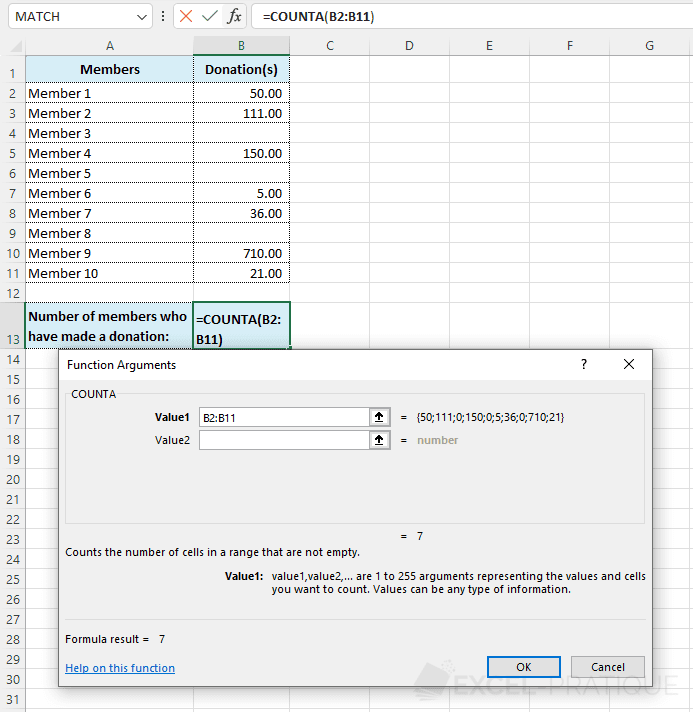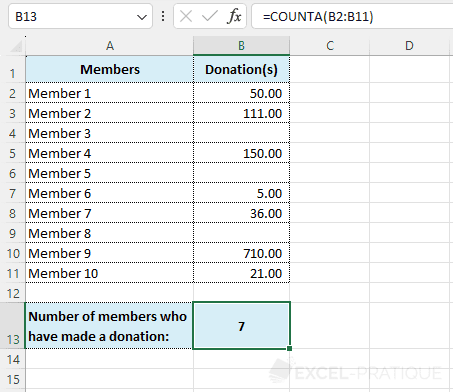# Excel Function: COUNTA

The Excel function COUNTA is used to determine the number of cells that are not empty.

Usage:

`=COUNTA(range_of_cells)`

## Example of use

The goal here is to get the total number of members who made a donation:Select the COUNTA function and then simply enter the range of cells in which to count the number of non-empty cells:

``=COUNTA(B2:B11)``Click OK to get the result: